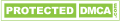# What is the Division Number of Thermocouple? What are the Characteristics?

Thermocouples are divided into S, R, B, N, K, E, J, T and so on. Among them, S, R and B belong to the precious metal thermocouple, N, K, E, J and T belong to the metal thermocouple.

The thermocouple of S is characterized by strong oxidation resistance, it should be used in oxidizing and inert atmosphere for continuous use, long-term use temperature of 1400 degrees, short-term use temperature of 1600 degrees. Of all thermocouples, the S scale has the highest degree of accuracy and is usually used as a standard thermocouple.

The R and S degrees are about 15 percent larger than the thermoelectric electromotive force, and the other performance is almost identical. The B index number is very small at room temperature, so it is not necessary to compensate the lead in the measurement. Its long using temperature is 1600 ℃, and short-term using temperature is 1800 ℃. It can be used in oxidizing or neutral atmosphere, and can be used in short term in vacuum condition.

N scoring number is characterized by high temperature resistance to oxidation at 1300 degrees Celsius. The long-term stability of thermoelectric electromotive force and the recovery of short-term heat cycle are good, it is also good at nuclear radiation and low temperature resistance, it can be substituted for the s-scale thermocouple; The K index number is characterized by strong antioxidant properties, it is appropriate to use in oxidation, the inert atmosphere, and its long-term using temperature is 1000 ℃, and short-term using temperature is 1200 ℃, It is used most widely in all thermocouples; The characteristic of E scale is that its thermoelectric force is the largest and it is the most sensitive. It should be used continuously in oxidizing and inert atmosphere with a temperature of 0-800 ; The J scale is characterized by its use in oxidizing atmospheres (upper temperature limit of 750 degrees),and it can also be used for reducing atmosphere (using the upper limit of temperature 950 ℃), and it is resistant to corrosion gas H2 and CO, it is widely used in oil refining and chemical engineering; The T scale is characterized by the highest level of accuracy in all low metal thermocouples, it is usually used to measure the temperatures below 300 degrees Celsius.

Related Articles